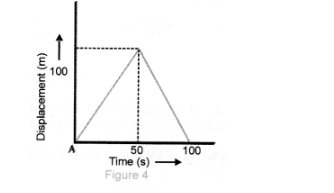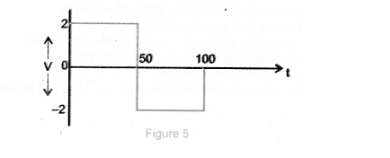# A girl walks along a straight path to drop`
Question:

A girl walks along a straight path to drop a letter in the letterbox and comes back to her initial position. Her displacement-time graph is shown in Fig. 4. Plot a velocity-time graph for the same.Solution:

For 0 to $50 \mathrm{~s}, \mathrm{v}=\frac{100}{50}=2 \mathrm{~m} \mathrm{~s}^{-1}$

For $50 s$ to $100 s, v=\frac{-100}{50}=-2 \mathrm{~m} \mathrm{~s}^{-1}$. Velocity-time graph is shown in figure 5 .